Courses

# Olympiad Test: Odd One Out - 1

## 10 Questions MCQ Test Mathematics Olympiad Class 7 | Olympiad Test: Odd One Out - 1

Description
This mock test of Olympiad Test: Odd One Out - 1 for Class 7 helps you for every Class 7 entrance exam. This contains 10 Multiple Choice Questions for Class 7 Olympiad Test: Odd One Out - 1 (mcq) to study with solutions a complete question bank. The solved questions answers in this Olympiad Test: Odd One Out - 1 quiz give you a good mix of easy questions and tough questions. Class 7 students definitely take this Olympiad Test: Odd One Out - 1 exercise for a better result in the exam. You can find other Olympiad Test: Odd One Out - 1 extra questions, long questions & short questions for Class 7 on EduRev as well by searching above.
QUESTION: 1

### Direction: In each problem, out of the five figures marked (1), (2), (3), (4) and (5), four are similar in a certain manner. However, one figure is not like the other four.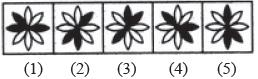Q. Choose the figure which is different from the rest.

Solution:

All other figures can be rotated into each other.

QUESTION: 2

### Direction: In each problem, out of the five figures marked (1), (2), (3), (4) and (5), four are similar in a certain manner. However, one figure is not like the other four. Q. Choose the figure which is different from the rest.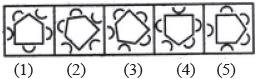Solution:

In each one of the figures except figure (2), three cups open towards the pentagon and two cups open outwards.

QUESTION: 3

### Direction: In each problem, out of the five figures marked (1), (2), (3), (4) and (5), four are similar in a certain manner. However, one figure is not like the other four. Q. Choose the figure which is different from the rest.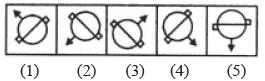Solution:

All other figures can be rotated into each other.

QUESTION: 4

Direction: In each problem, out of the five figures marked (1), (2), (3), (4) and (5), four are similar in a certain manner. However, one figure is not like the other four.
Q. Choose the figure which is different from the rest.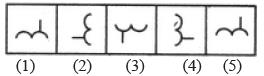Solution:

All other figures can be rotated into each other.

QUESTION: 5

Direction: In each problem, out of the five figures marked (1), (2), (3), (4) and (5), four are similar in a certain manner. However, one figure is not like the other four.
Q. Choose the figure which is different from the rest.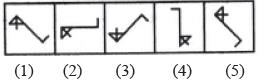Solution:

The inclination of the triangle is different in figure (4).

QUESTION: 6

Direction: In each problem, out of the five figures marked (1), (2), (3), (4) and (5), four are similar in a certain manner. However, one figure is not like the other four.
Q. Choose the figure which is different from the rest.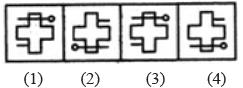Solution:

Only in figure (4), the small line segment lies on the same side (right side) of the main figure as the head of the pin (or all other figures can be rotated into each other).

QUESTION: 7

Direction: In each problem, out of the five figures marked (1), (2), (3), (4) and (5), four are similar in a certain manner. However, one figure is not like the other four.
Q. Choose the figure which is different from the rest.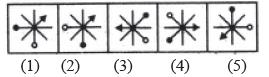Solution:

All other figures can be rotated into each other.

QUESTION: 8

Direction: In each problem, out of the five figures marked (1), (2), (3), (4) and (5), four are similar in a certain manner. However, one figure is not like the other four.
Q. Choose the figure which is different from the rest.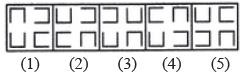Solution:

Only in figure (3), two out of the four elements are opening in the same direction.

QUESTION: 9

Direction: In each problem, out of the five figures marked (1), (2), (3), (4) and (5), four are similar in a certain manner. However, one figure is not like the other four.
Q. Choose the figure which is different from the rest.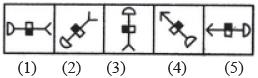Solution:

If all the figures are rotated so that the semicircle lies on the top, then the shaded part of the rectangle lies on the LHS.

QUESTION: 10

Direction: In each problem, out of the five figures marked (1), (2), (3), (4) and (5), four are similar in a certain manner. However, one figure is not like the other four.
Q. Choose the figure which is different from the rest.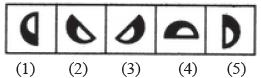Solution:

All other figures can be rotated into each other.